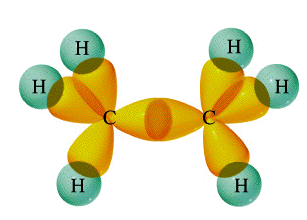# Question #b497b

Jan 27, 2014

To count sigma and pi bonds, first you need to know how many single, double and triple bonds are present in the molecule.

#### Explanation:

A single bond contains one sigma bond (direct head-on overlap of orbitals).For example, the $\text{C-C}$ sigma bond in ethane is formed by the head-on overlap of two $s {p}^{3}$ orbitals. The C-H sigma bonds are formed by $s \text{-} s {p}^{3}$ overlap.

A double bond contains one sigma bond and one pi bond (side-on overlap of $p$ orbitals).In ethylene, the $\text{C=C}$ double bond consists of a sigma bond and a pi bond. The sigma bond is formed by the head-on overlap of two $s {p}^{2}$ orbitals. The pi bond is formed by the side-on overlap of two $2 p$ orbitals.

A triple bond contains one sigma bond and 2 pi bonds.In acetylene, the $\text{C≡C}$ triple bond consists of a sigma bond and two pi bonds. The sigma bond is formed by the head-on overlap of two $s p$ orbitals. The pi bonds are formed by the side-on overlap of $2 p$ orbitals.

It's just that simple.

Here's a video on counting sigma and pi bonds.